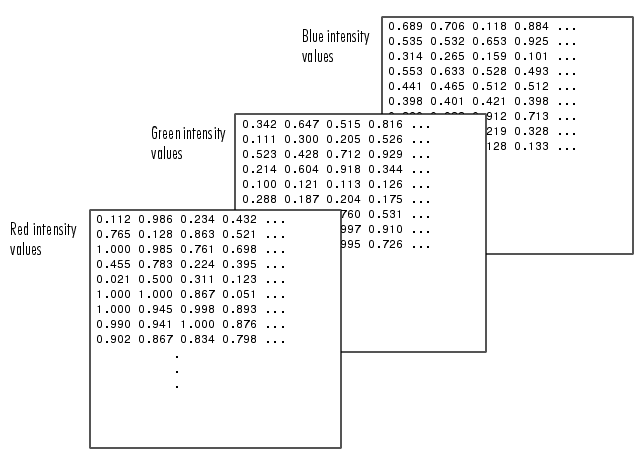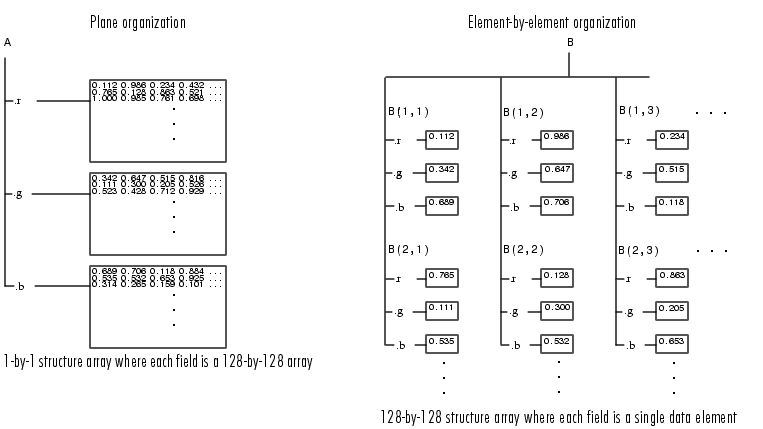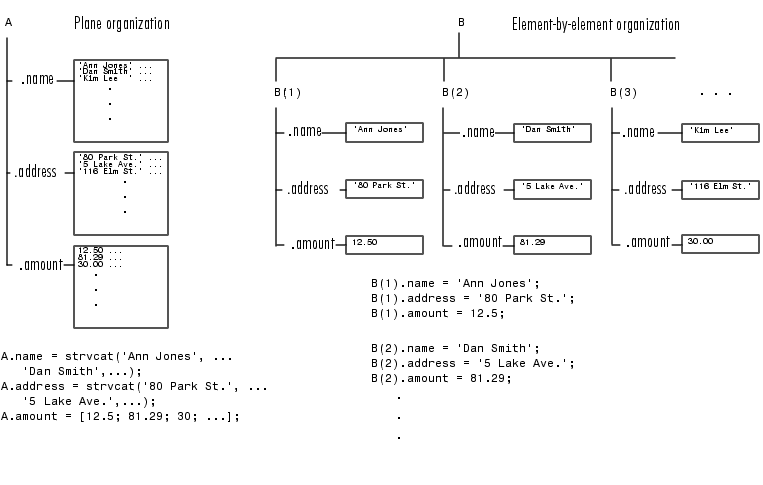ProgrammingOrganizing Data in Structure Arrays

The key to organizing structure arrays is to decide how you want to access subsets of the information. This, in turn, determines how you build the array that holds the structures, and how you break up the structure fields.

For example, consider a 128-by-128 RGB image stored in three separate arrays; `RED`, `GREEN`, and `BLUE`.There are at least two ways you can organize such data into a structure array.Plane Organization

In the plane organization, shown to the left in the figure above, each field of the structure is an entire plane of the image. You can create this structure using

• ```A.r = RED;
A.g = GREEN;
A.b = BLUE;
```

This approach allows you to easily extract entire image planes for display, filtering, or other tasks that work on the entire image at once. To access the entire red plane, for example, use

• ```redPlane = A.r;
```

Plane organization has the additional advantage of being extensible to multiple images in this case. If you have a number of images, you can store them as `A(2)`, `A(3)`, and so on, each containing an entire image.

The disadvantage of plane organization is evident when you need to access subsets of the planes. To access a subimage, for example, you need to access each field separately:

• ```redSub = A.r(2:12,13:30);
greenSub = A.g(2:12,13:30);
blueSub = A.b(2:12,13:30);
```

Element-by-Element Organization

The element-by-element organization, shown to the right in the figure above, has the advantage of allowing easy access to subsets of data. To set up the data in this organization, use

• ```for m = 1:size(RED,1)
for n = 1:size(RED,2)
B(m,n).r = RED(m,n);
B(m,n).g = GREEN(m,n);
B(m,n).b = BLUE(m,n);
end
end
```

With element-by-element organization, you can access a subset of data with a single statement:

• ```Bsub = B(1:10,1:10);
```

To access an entire plane of the image using the element-by-element method, however, requires a loop:

• ```redPlane = zeros(128, 128);
for k = 1:(128 * 128)
redPlane(k) = B(k).r;
end
```

Element-by-element organization is not the best structure array choice for most image processing applications; however, it can be the best for other applications wherein you will routinely need to access corresponding subsets of structure fields. The example in the following section demonstrates this type of application.

Example -- A Simple Database

Consider organizing a simple database.Each of the possible organizations has advantages depending on how you want to access the data:

• Plane organization makes it easier to operate on all field values at once. For example, to find the average of all the values in the `amount` field,
• - Using plane organization

• ```    avg = mean(A.amount);
```

- Using element-by-element organization

• ```    avg = mean([B.amount]);
```
• Element-by-element organization makes it easier to access all the information related to a single client. Consider an M-file, `client.m`, which displays the name and address of a given client on screen.
• Using plane organization, pass individual fields.

• ```  function client(name,address)
disp(name)
disp(address)
```

To call the `client` function,

• ```  client(A.name(2,:),A.address(2,:))

```

Using element-by-element organization, pass an entire structure.

• ```  function client(B)
disp(B)
```

To call the `client` function,

• ```  client(B(2))
```
• Element-by-element organization makes it easier to expand the string array fields. If you do not know the maximum string length ahead of time for plane organization, you may need to frequently recreate the `name` or `address` field to accommodate longer strings.

Typically, your data does not dictate the organization scheme you choose. Rather, you must consider how you want to access and operate on the data.Writing Functions to Operate on Structures Nesting Structures© 1994-2005 The MathWorks, Inc.